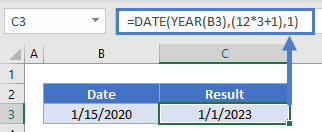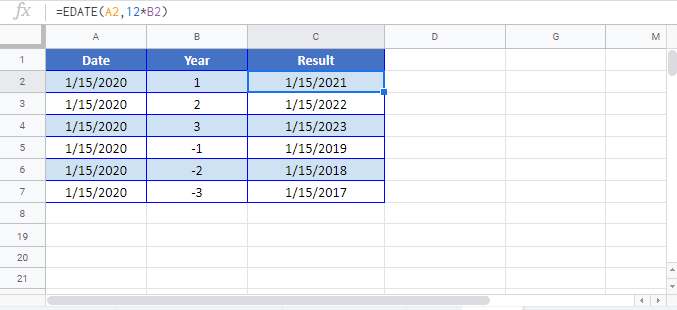# Add or Subtract Years to a Date in Excel & Google Sheets

This tutorial will demonstrate how to add (or subtract) years to a date in Excel and Google Sheets.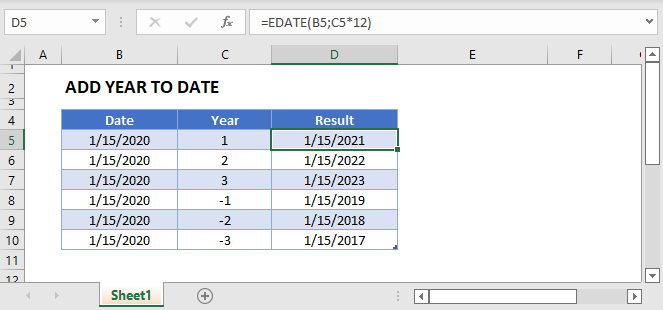## Add Years to a Date

The easiest way to add years to a date is with the EDATE Function. The EDATE Function adds a specified number of months to a date. By adding months in multiplies of 12 (12,24,36, etc.) you can add years to a date.

### Add 1 Year to a Date

This example will add 1 year to a date:

``=EDATE(B3,12)``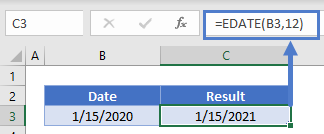### Add 5 Years to a Date

This example will add 5 years to a date:

``=EDATE(B3,12*5)``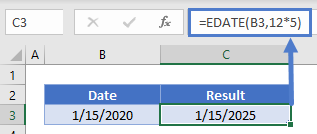## Subtract Years From a Date

To subtract years from a date, simply enter a negative number of months into the EDATE Function:

``=EDATE(B3,-12*3)``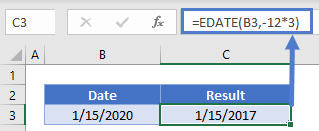## Add Years with DATE Function

Another way to add years to a date is with the YEAR, MONTH, DAY and DATE Functions:

``=DATE(YEAR(B3),(12*3+MONTH(B3)),DAY(B3))``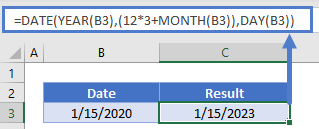The DATE Function creates a date using a specified Year, Month, and Day. We populate the DATE function with the current date’s year, month + a number of months and day using the YEAR, MONTH, and DAY Functions.

### Subtract Years with DATE Function

Of course the same logic applies with subtracting dates:

``=DATE(YEAR(B3),(-12*5+MONTH(B3)),DAY(B3))``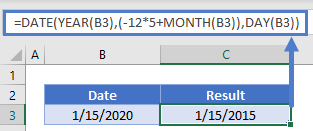### Easy Excel Automation### Add Years – First Day of New Year

To add years to a date and return the first day of the calculated year, you can also use the DATE Function:

``=DATE(YEAR(B3),(12*3+1),1)``